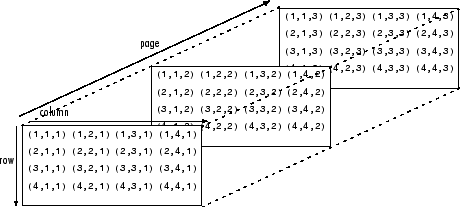# pageinv

Page-wise matrix inverse

Since R2022a

## Syntax

``Y = pageinv(X)``
``[Y,RC] = pageinv(X)``

## Description

example

````Y = pageinv(X)` computes the inverse of each page of N-D array `X`. Each page of the output array `Y` is given by `Y(:,:,i) = inv(X(:,:,i))`.If `X` has more than three dimensions, then `pageinv` returns an N-D array with the same dimensions, as in `Y(:,:,i,j,k) = inv(X(:,:,i,j,k))`.```

example

````[Y,RC] = pageinv(X)` also returns the reciprocal condition number of each page of `X`. The value of `RC(1,1,i)` is the reciprocal of the condition number of `X(:,:,i)`. If `RC(1,1,i) < eps`, then the call `inv(X(:,:,i))` returns a warning that `X(:,:,i)` is ill conditioned. However, `pageinv` does not issue a warning for ill-conditioned inputs.```

## Examples

collapse all

Create a 3-by-3-by-3 array by concatenating several 3-by-3 matrices.

`X = cat(3,magic(3),hilb(3),pascal(3))`
```X = X(:,:,1) = 8 1 6 3 5 7 4 9 2 X(:,:,2) = 1.0000 0.5000 0.3333 0.5000 0.3333 0.2500 0.3333 0.2500 0.2000 X(:,:,3) = 1 1 1 1 2 3 1 3 6 ```

Calculate the matrix inverse of each array page.

`Y = pageinv(X)`
```Y = Y(:,:,1) = 0.1472 -0.1444 0.0639 -0.0611 0.0222 0.1056 -0.0194 0.1889 -0.1028 Y(:,:,2) = 9.0000 -36.0000 30.0000 -36.0000 192.0000 -180.0000 30.0000 -180.0000 180.0000 Y(:,:,3) = 3.0000 -3.0000 1.0000 -3.0000 5.0000 -2.0000 1.0000 -2.0000 1.0000 ```

The `i`th page of the output, `Y(:,:,i)`, is found by calculating the matrix inverse `Y(:,:,i) = inv(X(:,:,i))`.

Create a 10-by-10-by-2 array with a magic square matrix on the first page and a Hilbert matrix on the second page.

`X = cat(3,magic(10),hilb(10))`
```X = X(:,:,1) = 92 99 1 8 15 67 74 51 58 40 98 80 7 14 16 73 55 57 64 41 4 81 88 20 22 54 56 63 70 47 85 87 19 21 3 60 62 69 71 28 86 93 25 2 9 61 68 75 52 34 17 24 76 83 90 42 49 26 33 65 23 5 82 89 91 48 30 32 39 66 79 6 13 95 97 29 31 38 45 72 10 12 94 96 78 35 37 44 46 53 11 18 100 77 84 36 43 50 27 59 X(:,:,2) = 1.0000 0.5000 0.3333 0.2500 0.2000 0.1667 0.1429 0.1250 0.1111 0.1000 0.5000 0.3333 0.2500 0.2000 0.1667 0.1429 0.1250 0.1111 0.1000 0.0909 0.3333 0.2500 0.2000 0.1667 0.1429 0.1250 0.1111 0.1000 0.0909 0.0833 0.2500 0.2000 0.1667 0.1429 0.1250 0.1111 0.1000 0.0909 0.0833 0.0769 0.2000 0.1667 0.1429 0.1250 0.1111 0.1000 0.0909 0.0833 0.0769 0.0714 0.1667 0.1429 0.1250 0.1111 0.1000 0.0909 0.0833 0.0769 0.0714 0.0667 0.1429 0.1250 0.1111 0.1000 0.0909 0.0833 0.0769 0.0714 0.0667 0.0625 0.1250 0.1111 0.1000 0.0909 0.0833 0.0769 0.0714 0.0667 0.0625 0.0588 0.1111 0.1000 0.0909 0.0833 0.0769 0.0714 0.0667 0.0625 0.0588 0.0556 0.1000 0.0909 0.0833 0.0769 0.0714 0.0667 0.0625 0.0588 0.0556 0.0526 ```

Calculate the matrix inverse of each array page. Specify two outputs to also return the reciprocal condition number of each matrix that is being inverted.

```[Y,RC] = pageinv(X); RC```
```RC = RC(:,:,1) = 3.0812e-18 RC(:,:,2) = 2.8287e-14 ```

Compare the reciprocal condition numbers to `eps`. The results indicate that the magic square matrix is ill conditioned, so the results of the inversion operation for that page are not reliable.

`RC < eps`
```ans = 1x1x2 logical array ans(:,:,1) = 1 ans(:,:,2) = 0 ```

Check the results by calculating the residual norm between `X(:,:,i)*Y(:,:,i)` and the identity matrix. The large residual norm for the first page confirms that the matrix inverse for that page is not accurate.

`norm(X(:,:,1)*Y(:,:,1) - eye(10))`
```ans = 23.5370 ```
`norm(X(:,:,2)*Y(:,:,2) - eye(10))`
```ans = 1.2258e-04 ```

## Input Arguments

collapse all

Input array, specified as a matrix or multidimensional array.

Data Types: `single` | `double`
Complex Number Support: Yes

## Output Arguments

collapse all

Page-wise inverses, returned as a multidimensional array. `Y` has the same size and data type as `X`. Each page `Y(:,:,i,...)` is the matrix inverse of `X(:,:,i,...)`.

Reciprocal condition numbers, returned as a multidimensional array with the same number of pages as `X`. The value of `RC(1,1,i)` is the reciprocal of the condition number of `X(:,:,i)`. If `RC(1,1,i) < eps`, then the call `inv(X(:,:,i))` returns a warning that `X(:,:,i)` is ill conditioned. However, `pageinv` does not issue a warning for ill-conditioned inputs.

collapse all

### Array Pages

Page-wise functions like `pageinv` operate on 2-D matrices that have been arranged into a multidimensional array. For example, with a 3-D array the elements in the third dimension of the array are commonly called pages because they stack on top of each other like pages in a book. Each page is a matrix that gets operated on by the function.You can also assemble a collection of 2-D matrices into a higher dimensional array, like a 4-D or 5-D array, and in these cases `pageinv` still treats the fundamental unit of the array as a 2-D matrix that gets operated on, such as `X(:,:,i,j,k,l)`.

The `cat` function is useful for assembling a collection of matrices into a multidimensional array, and the `zeros` function is useful for preallocating a multidimensional array.

## Tips

• Results obtained using `pageinv` are numerically equivalent to computing the inverses of each of the same matrices in a `for`-loop. However, the two results might differ slightly due to floating-point round-off error.

## Version History

Introduced in R2022a